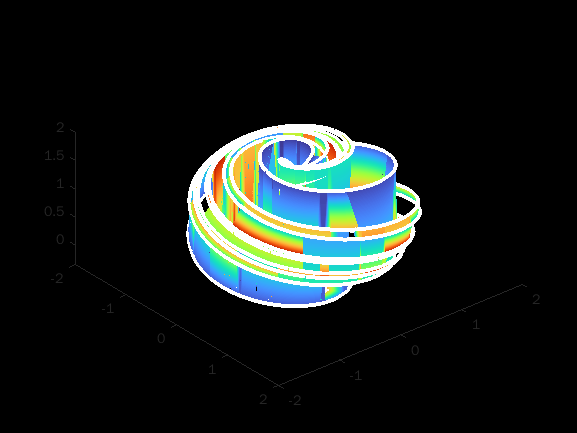• /
• # The Aizawa's Attractor

on 11 Oct 2021
• 31
• 295
• 4
• 0
• 280
%% AIZAWA ACTTRACTOR
T = 50;
t = 0:5e-5:T;
%% solving chaotic dynamics
[~,y]=ode15s(@f,t,[.1 0 0]);
patch(y(:,1),y(:,2),y(:,3),t,'EdgeC','w','Linew',3);
%% plotting magic ;)
set([gcf,gca],'Color','k');
colormap(turbo);
view(T,T)%% odinary differential equation - Aizawa Attractor
function w = f(t,X)
x = X(1);
y = X(2);
z = X(3);
d = 3.5;
b = z-.7;
w = [b*x-d*y; d*x+b*y; .6 + .95*z - z^3/3 - (x^2+y^2)*(1+z/4) + .1*z*x^3];
end
%% References:
%  The Aizawa Attractor,
% https://www.algosome.com/articles/aizawa-attractor-chaos.html
% (Accessed on October 11, 2021).